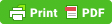# How to Calculate Cooling Capacity of a Chiller in HVAC (With PDF)

Chillers are extremely energy intensive to operate in the entire HVAC system of a building and that also means these are very expensive to run so, it is important to understand how efficient your chiller is operating.

We're going to be looking at how to calculate the chiller cooling capacity, and how much cooling can the chiller produce at any given time during its operation. Now there's going to be a few things we'll need to know in order to perform this calculation.

So let's imagine we've got our chiller here. We've got the return water that is coming from around the building that will return to water coming in the evaporator where it cools down and then leaves again and goes off through the building.

What is a Plenum Box & How to Size a Plenum Box for AHU

Before doing this calculation we need to know some important parameters like

Q = Heat Transfer Kw

Tin Water Temperature In Evaporator

Tout = Water Temperature Out Evaporator

Volume Flow Rate

ρ Density of Water

Cp = Specific Heat Capacity

The values of density of water and specific heat capacity of water will be taken at average temperature = (Tin+ Tout)/2

To find the values you can simply look them up in your engineering tables in books, or you go here to find the values.

We're going to assume that

Volume Flow Rate = 0.0995 m3/s

Water Temperature In Tin = 12 ˚C

Water Temperature Out Tout = 6 ˚C

Average Temperature = (Tin+ Tout)/2 = (12+6)/2 = 9 ˚C

For density and specific heat capacity at average go to the above link and put to values of working pressure and average temperature i.e.

Pressure P = 101.325 kPa

Average Temperature = 9˚C

You will get

Density of Water ρ = 999.78 kg/m3

Specific Heat Capacity Cp = 4.19 kj/kg.K

Now Formula for the for the heat transfer is

Q = ( x ρ ) x Cp x (Tin - Tout )

Where V̇ x ρ = Mass Flow Rate

Now put the values in the above equation

Q = (0.0995 m3/s x 999.78 kg/m3) x 4.19 kj/kg.K x ((12˚C +273.15K) – (6˚C + 273.16K))

Note: 273.16K is added in the temperature to convert Celsius into Kelvin.

Simplifying above equation:

Q = 99.48 kg/s x 4.19 kj/kg.K x (285.15K279.15K)

Q = 99.48 kg/s x 4.19 kj/kg.K x 6K

Q = 2500 kW (Refrigeration effect)

To convert it into Ton of Refrigeration divide it by 3.517

Q = 2500 kW / 3.517

Q = 711 TR Refrigeration Tons

To convert it into BTU/h multiply above value with 3412.142

Q = 2500 kW x 3412.145

Q = 853357 BTU/h

So that is how you calculate your cooling capacity of the chiller and you can apply this any time during its operation, whether it's a part load or a full load, it will be calculated like this. If you have any comments, leave them in the comment section down below.